# Math in Focus Grade 2 Cumulative Review for Chapters 10 to 12 Answer Key

Practice the problems of Math in Focus Grade 2 Workbook Answer Key Cumulative Review for Chapters 10 to 12 to Estimate to score better marks in the exam.

## Math in Focus Grade 2 Cumulative Review for Chapters 10 to 12 Answer Key

Concepts and Skills

Question 1.
Connect the cards to show the steps for mental math.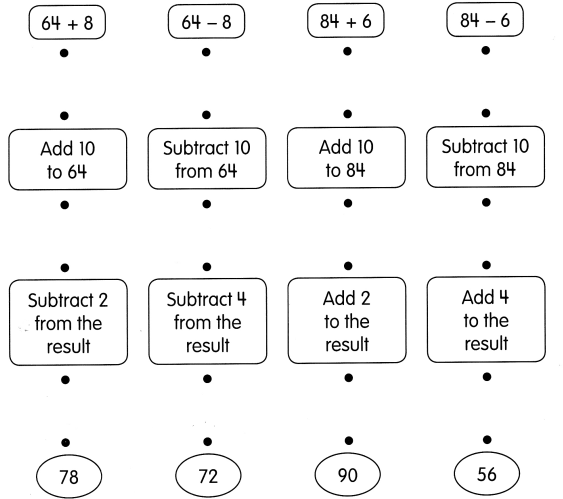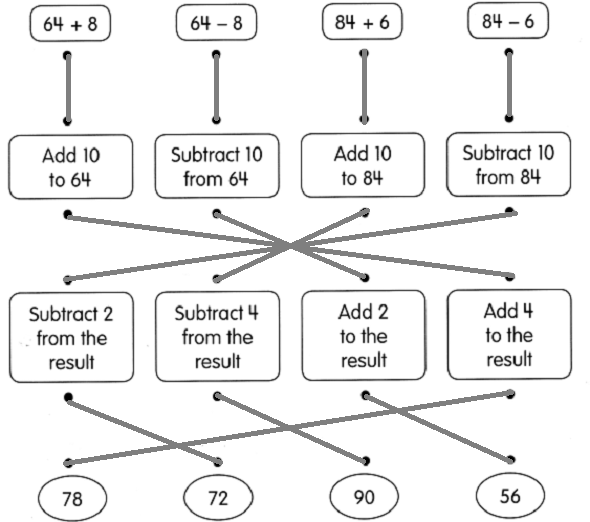Question 2.
352 + 4 = ____________
Answer: 352 + 4 = 356

Question 3.
817 + 5 = ____________
Answer: 817 + 5 = 822

Question 4.
143 + 30 = ____________
Answer: 143 + 30 = 163

Question 5.
198 + 800 = ____________
Answer: 198 + 800 = 998

Subtract mentally.

Question 6.
916 – 5 = ____________
Answer: 916 – 5 = 911

Question 7.
873 – 8 = ____________
Answer: 873 – 8 = 865

Question 8.
477 – 60 = ____________
Answer: 477 – 60 = 416

Question 9.
858 – 400 = ____________
Answer: 858 – 400 = 458

Mark each number with an X on the number line. Then round each number to the nearest ten.

Question 10.
76
Answer: 76 is nearer to 80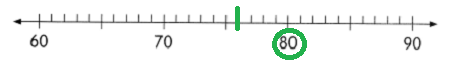Question 11.
81
Answer: 81 is nearest to 80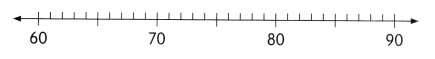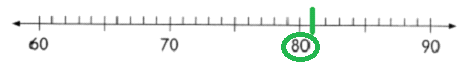Question 12.
123
123 is nearest to 120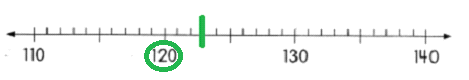Question 13.
134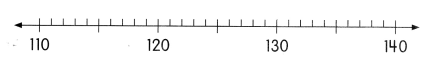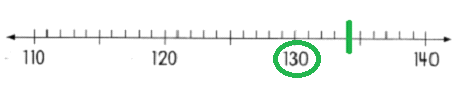Complete.

Question 14.
Write the numbers that give 50 when rounded to the nearest ten.
The numbers that give 50 when rounded to the nearest ten are 45,46,47,48,49,51,52,53,54.

Question 15.
What is the least number that rounds to 10?
Answer: The least number that rounds to 10 is 1.

Question 16.
What is the greatest number that rounds to 80?
Answer: The greatest number that rounds to 80 is 84.

Add or subtract. Round each number to the nearest ten. Then estimate the sum or difference to check that your answer is reasonable.

Question 17.
874 + 67 = ___________
____________ + ____________ = ____________
So, 874 + 67 is about ____________
874 + 67 = 941
874 is about nearest to 870
67 is about nearest to 70
870 + 70 = 940
So, 874 + 67 is about nearest to 941

Question 18.
545 – 79 = ____________
So, 545 – 79 is about ____________
545 – 79 = 466
545 is about nearest to 550
79 is about nearest to 80
So, 545 – 79 is about 466

Circle the bills that make the amount shown.

Question 19.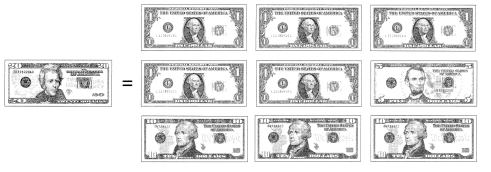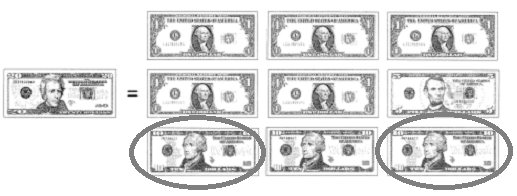Write the amount in numbers.

Question 20.
twenty-five cents $__________ or __________¢ Answer:$0.25

Question 21.
thirty-nine dollars $____________ Answer:$39

Question 22.
twelve dollars and ninety-seven cents $____________ Answer:$12.97

Count the money. Then write the amount each way.

Question 23.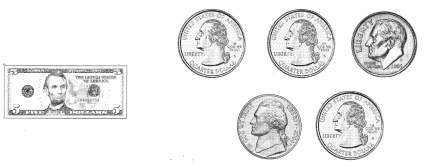dollars and cents ____________
cents ____________
words ____________
dollars and cents $5.90 cents 90 words: Five dollars and 90 cents Circle the amount that is least. Question 24.$10.75
$7.98$8.07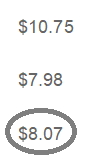Circle the greatest amount.

Question 25.
$96.50$96.72
$96.09 Answer: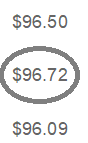Shade the model to show the fraction. Question 26.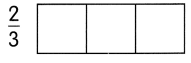Answer: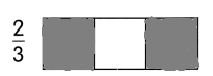Question 27.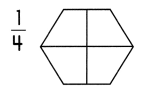Answer: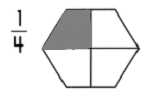Look at the model.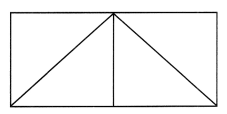Color $$\frac{1}{4}$$ blue. Color $$\frac{2}{4}$$ yellow. Question 28. What fraction of the model is colored? Answer: 1/4 fraction of the model is colored in blue.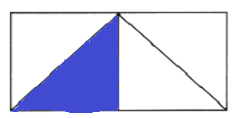Question 29. What fraction of the model is not colored? Answer: 2/4 fraction of the model is not colored in yellow.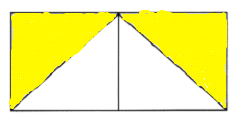Shade each strip. Then write the fractions in order from greatest to least. Question 30.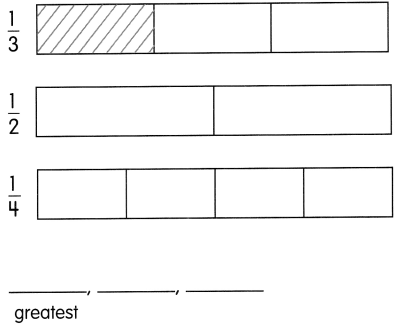Answer: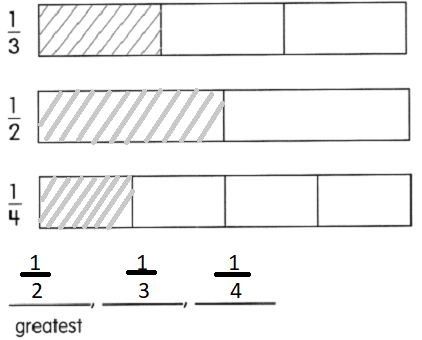Write a fraction for the shaded part. Question 31.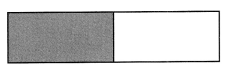Answer: 1/2 is the shaded part Question 32.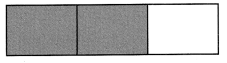Answer: 2/3 is the shaded part Question 33.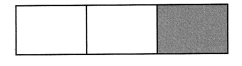Answer: 1/3 is the shaded part. Use your answers for Exercises 31 to 33. Fill in the blanks. Question 34. _______ is lout of 2 equal parts. Answer: 1/2 is 1 out of 2 equal parts. Question 35. _________ is 2 out of 3 equal parts. Answer: 2/3 is 2 out of 3 equal parts. Question 36. $$\frac{1}{2}$$ is greater than ___________. Answer: 1/2 is greater than 1/3. Question 37. $$\frac{1}{2}$$ is less than ______________. Answer: 1/2 is less than 1 Question 38. ______________ is the least fraction. Answer: Find the missing fraction. Use models to help you. Question 39. Add $$\frac{1}{3}$$ and $$\frac{1}{3}$$.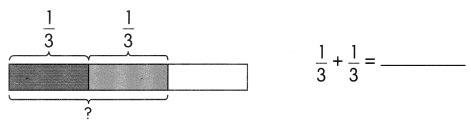Answer: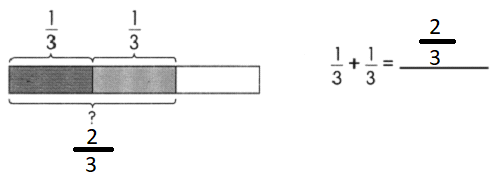Question 40. Subtract $$\frac{3}{4}$$ from 1.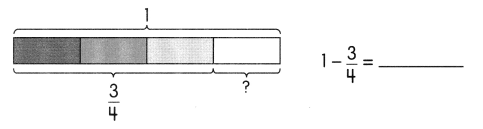Answer: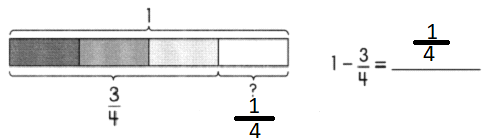Solve. Draw bar models to help you. Estimate to check your answers. Question 41. Teri folds 32 pieces of paper. Her sister folds 19 pieces. How many pieces do they fold in all? They fold ____________ pieces in all. Answer: They fold 51 pieces in all. Explanation: Given, Teri folds 32 pieces of paper, Her sister folds 19 pieces of paper, By adding 32 with 19 we get 51, Therefore, they fold 51 pieces of paper altogether. Question 42. Edwin has 83¢. His father gives him 25c more. How much does he have now? He has$__________ now.
Edwin has $1.08 now. Explanation: Given, Edwin has 83¢, His father gives him 25¢ more, By adding 83 with 25 we get 108¢, Converting 108¢ into dollars we get$1.08,
Therefore, Edwin has $1.08. Question 43. Jonas needs to deliver 34 newspapers. He still has 11 newspapers left to deliver. How many newspapers has he delivered? He has delivered ____________ newspapers. Answer: Jonas has delivered 23 newspapers. Explanation: Given, Jonas needs to deliver 34 newspapers, He still has 11 newspapers left to deliver, By subtracting 11 from 34 we get 23, Therefore, Jonas needs to deliver 23 newspapers. Question 44. Adam wants to buy a bat for$23 and a baseball glove for $17. He has saved$19. How much more money does he need?
He needs $____________ more. Answer: Adam needs$21 more.
Explanation:
Given,
Adam wants to buy bat for $23, And a baseball glove for$17,
He saved $19, By adding$23 with $17 we get$40 money which he needs to buy the things he want,
By subtracting $19 from$40 we get $21 money which he needs, Therefore, Adam needs$21 more to buy the things he want.

Question 45.
An eraser costs 16¢ and a pencil costs 70¢. Marian buys two erasers and a pencil. How much does she spend?
Marian spends $____________ Answer: Marian spends$1.02
Explanation:
Given,
An eraser costs 16¢,
Pencil costs 70¢,
Marian buys two erasers and a pencil,
By multiplying 2 with 16¢ we get the cost of 2 erasers which is 32¢,
By adding 32¢ with 70¢, we get the cost of 2 erasers and a pencil which is 102¢,
Converting 102¢ into dollars we get $1.02. Question 46. Mrs. Barry has$200 to buy new clothes. Round the cost of each item to the nearest ten. Then estimate the total cost.
A pair of pants costs $44. a. 44 is ____________ when rounded to the nearest ten. A pair of shoes costs$59.
A pair of socks costs $5. Answer: 59 is 60 when rounded to the nearest ten. c. 5 is ____________ when rounded to the nearest ten. A blouse costs$28.
Total cost is $____________ Answer: 28 is 30 when rounded to the nearest ten. Does Mrs. Barry have enough money to pay for all the items? Explain your answer. Answer: Total estimated cost is$140.
Mrs. Barry has $200 to buy new clothes, A pair of pants costs$44 rounded to $50, A pair of shoes costs$59 rounded to $60, A pair of socks costs$5 rounded to $10, A blouse costs$28 rounded to $30, Total cost of the clothes is by adding 44, 59, 5 and 28 we get$136,